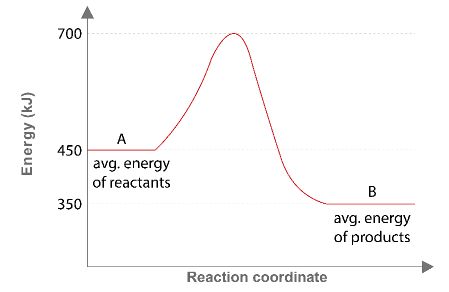# Problem: Consider the potential energy diagram below:What is the change in enthalpy (ΔH ) for the reaction A → B?a. -350 kJb. 100 kJc. -100 kJd. 350 kJ

###### FREE Expert Solution

We’re being asked to calculate the change in enthalpy (ΔH) for the reaction given the energy diagram

Recall that ΔH for a reaction is given by:###### Problem Details

Consider the potential energy diagram below:

What is the change in enthalpy (ΔH ) for the reaction A → B?

a. -350 kJ

b. 100 kJ

c. -100 kJ

d. 350 kJWhat scientific concept do you need to know in order to solve this problem?

Our tutors have indicated that to solve this problem you will need to apply the Energy Diagram concept. If you need more Energy Diagram practice, you can also practice Energy Diagram practice problems.

What is the difficulty of this problem?

Our tutors rated the difficulty ofConsider the potential energy diagram below:What is the chan...as low difficulty.

How long does this problem take to solve?

Our expert Chemistry tutor, Sabrina took 2 minutes and 4 seconds to solve this problem. You can follow their steps in the video explanation above.

What professor is this problem relevant for?

Based on our data, we think this problem is relevant for Professor Biberdorf's class at TEXAS.